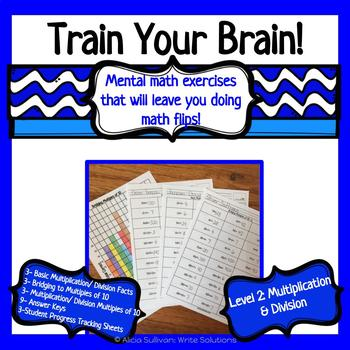# Multiplication and Division Fact PracticeSubject
Resource Type
File Type

PDF

(403 KB|15 pages)
Standards
• Product Description
• Standards
Helping students master their basic facts and gain valuable mental math strategies does not have take up lots of precious teaching time! It can be quick and student led!

I created this fact series when I was taking a Thinking Math class a few years back. The class emphasized the importance for students to be flexible with their use of operations; teaching addition and subtraction together and multiplication and division together.

My focus in creating these 15 practice sheets was to build students understanding of mental math and help them make connections between 7X8, 560/7 and 70x8. There are 5 sheets in each of three levels ultimately building up to completely mixed practice. Each practice sheet is organized in neat rows to easily and quickly facilitate whole class correction. There is also a place for the student corrector to record his/her initials or student #. In 10 minutes/ day for a few months, I have seen this series yield excellent results in grades 3,6 and 8.

Also FOLLOW ME to be the first to know when I post a new product. New products are 50% off for the first 24hours, so it pays to follow me!
Alicia:)
Find whole-number quotients of whole numbers with up to four-digit dividends and two-digit divisors, using strategies based on place value, the properties of operations, and/or the relationship between multiplication and division. Illustrate and explain the calculation by using equations, rectangular arrays, and/or area models.
Fluently multiply multi-digit whole numbers using the standard algorithm.
Find whole-number quotients and remainders with up to four-digit dividends and one-digit divisors, using strategies based on place value, the properties of operations, and/or the relationship between multiplication and division. Illustrate and explain the calculation by using equations, rectangular arrays, and/or area models.
Multiply a whole number of up to four digits by a one-digit whole number, and multiply two two-digit numbers, using strategies based on place value and the properties of operations. Illustrate and explain the calculation by using equations, rectangular arrays, and/or area models.
Total Pages
15 pages
Not Included
Teaching Duration
30 minutes
Report this Resource to TpT
Reported resources will be reviewed by our team. Report this resource to let us know if this resource violates TpT’s content guidelines.